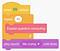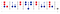# Quantum Algorithmic Structures

Welcome to Introduction to Quantum Computing. I am your guide, Associate Professor Chris Ferrie, a researcher in the UTS Centre for Quantum Software and Information. This is Lecture 8. It would probably be a good idea to have read the previous Lectures before continuing.

`What did you learn last week?Last week you were introduced to the Deutsch-Jozsa algorithm, which was the first computational algorithm to show a speed-up over any classical algorithm. You also learned about how to perform digital logic in a quantum computation and create quantum oracles.What will you learn this week?This week you will learn about the high-level structure of quantum algorithms and some primitives such as superposition, digital logic, phase logic, and uncomputation. With these you’ll at least be able to fake it until you make it!What will you be able to do at the end of this week 5?At the end of this module, you should be able to answer these questions:What is uncomputation?What are the core primitives of quantum algorithms?What is the basic structure of a quantum algorithm?`

# Would you recognise a quantum computation if you saw it?

What does an algorithm look like? First, there is no correct answer here. Algorithms can be written and visualised in many ways, which primarily depend on the audience, but also on what the model of computation and programming language is. For example, here is a Scratch program.Have a go at https://scratch.mit.edu/.

All Scratch programs — at least the basic ones being taught to kids — have this same structure: wait for start, follow a bunch of steps, then stop running. A simultaneous top-down and bottom-up approach is one where the high-level structure provides the holes the fundamental pegs fit into. And we can do the same thing for quantum programming.

The high-level structure of a quantum algorithm is as follows.

We’re generalising a bit when we say that all of these components are necessary. However, given only what we have seen so far in class, we can make some simple arguments for why each is necessary and what purpose they serve.

Let’s start with superposition. Without superposition we can never create entanglement. This means each qubit can be thought of as a distinct entity and describing them with classical information is efficient. Basis states will be mapped to basis states. Perhaps a global phase will be added, but we know these do not affect the outcomes of measurements. We need superpositions.

Next we perform some computation. This typically is where entanglement is generated. A simple example we have seen many times is the H+CNOT circuit. Again, entanglement is necessary to avoid building a circuit that can be classically simulated efficiently. Often we can distinguish this step from the next which creates relative phases. These are necessary to achieve interference between basis states. A large entangled state is not useful because a measurement of each qubit will generate random (though correlated) outcomes. We want to steer as close as possible toward deterministic outcomes, and this is achieved with phase manipulation.

At this point we have generated the answer, but it is entangled with other qubits. We need to disentangle to extract the answer. This comes in two steps. The first a copy-like operation that stores the answer in a new output register. The second step is the uncomputation which inverts everything to get back to the original state in all registers but the output register.

# Computing in superposition

This component of our quantum program is the most familiar. What we haven’t seen are many examples of digital logic encoded in quantum circuits. So let’s get some intuition for how that works.

You’ll recall that we had the following quantum logic circuits.

And we can check that these perform computation in superposition. For example:

Something we glossed over is the apparent necessity of extra qubits. Indeed, since AND, for example, is not a reversible gate, it cannot be implemented by a unitary operation on 2 qubits. Classically, we can turn any irreversible operation into a reversible one, and this requires adding more scratch bits. Nearly all quantum circuits contain these scratch qubits, which you’ll also see called work qubits or ancillary qubits.

Of course, if we measured after any of these superposition computations, we would get a random outcome. That’s not all that useful unless you wanted to create random numbers.

# Just a phase this state will grow out of

Recall that in the Deutsch-Jozsa circuit, the phase oracle was used to create interference. The balanced function output created phases that were completely cancelled out, whereas a constant function had phases which added up to a certain outcome returning to the starting state.

Above we looked at computing digital logic functions and storing the output in some work register. These are implementations of quantum oracles — called Boolean oracles — as we defined them in the previous lecture.

But in the Deutsch-Jozsa algorithm we need the phase. That is, we only needed an oracle performing the operation.

There isn’t a contradiction. Indeed, there is a direct correspondence between Boolean and phase oracles.

The Deutsch-Jozsa algorithm makes it obvious that superposition alone does not generate a useful resource in quantum computation. We also need interference — though at this point you might notice that complex numbers have yet to appear at all!

At this point we may have coordinated a beautiful computation, but the answer is still wrapped up in superposition. In the Deutsch-Jozsa algorithm we undid the superposition to return to a basis state which provided a deterministic answer. This undoing appears in one form or another in almost every quantum algorithm. Let’s see why.

# Uncompute but not uncomputable

Recall the Toffoli implementation of the AND gate above. We can similarly implement the AND of three variables.

However, if we wanted to perform this with only Tofolli gates, we would need an extra scratch qubit.Play with this circuit on Quirk.

You will note however that the last register is left in an unwanted state — technically called garbage. This is an issue if we want to perform computation in superposition. You don’t want to be entangled with garbage — not literally nor figuratively.

The fix is to uncompute (literally invert) the operations which modify the garbage qubits. In the above example it looks like this.Play with this circuit on Quirk.

In general we might have a computation where the garbage is not obviously invertible independent of the output. In that case, since the calculation is “classical”, we can copy the output to another final output register and then uncompute everything we had done prior to that. Suppose we had some new oracle which produced the output, but also some garbage we could not invert alone. In that case, our uncomputation looks like this.The full uncomputed circuit of the CCCNOT gate can be found on Quirk.

You’ll see these kinds of symmetric circuits everywhere. In the Deutsch-Jozsa circuit, the uncomputation was luckily not necessary to get the answer we were looking for. However, even when doing an experiment, it is much easier to perform a little uncomputation if only to easily interpret the answer. This is especially true in noisy devices. Below is the circuit I ran on the IBM quantum computer ibmq_16_melbourne.My IMBQ implementation of an uncomputed Deutsch-Jozsa circuit. You can also play around this circuit in simulation on Quirk.

Can you recognise it? I’ve copied the answer to a new output register. The zero outcome should now correspond to a balanced function. Indeed this oracle implements a balanced function and the IBM quantum computer gets the answer correct 65% of the time. Not bad!

# Additional Resources

Microsoft’s Quantum Katas contains katas on truth tables and on constructing the ripple carry adder.

Scott Aaronson’s blog post on complex numbers in quantum computation.

You can sign-up and run your own circuits on a real quantum computer at the IBM Quantum Experience.

Quantum theorist by day, father by night. Occasionally moonlights as a children’s book author. www.csferrie.com @csferrie

## More from Chris Ferrie

Quantum theorist by day, father by night. Occasionally moonlights as a children’s book author. www.csferrie.com @csferrie